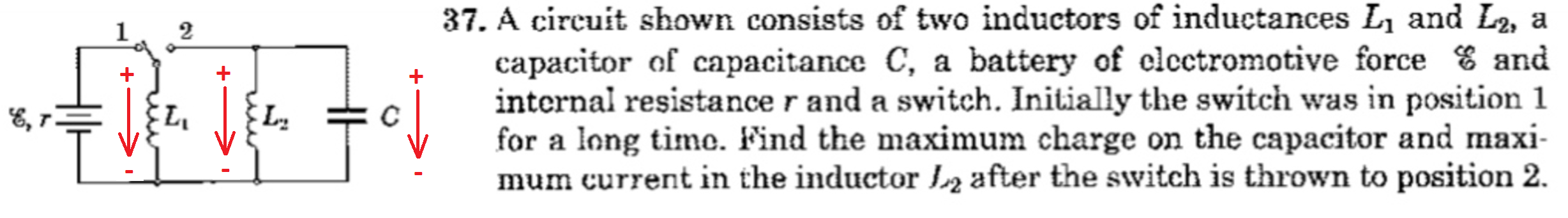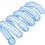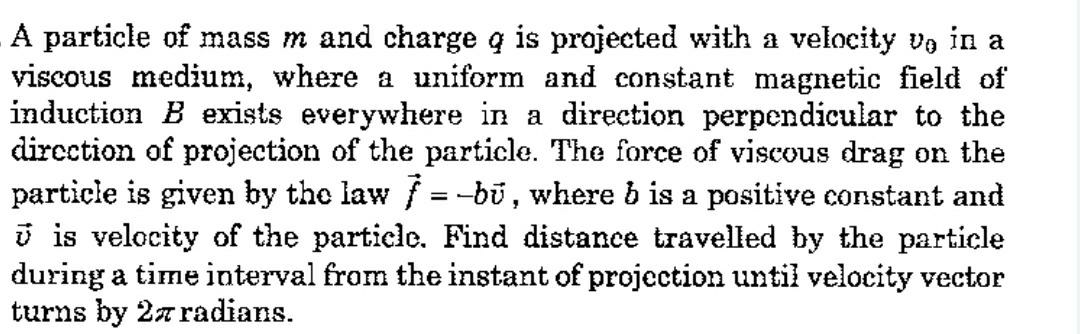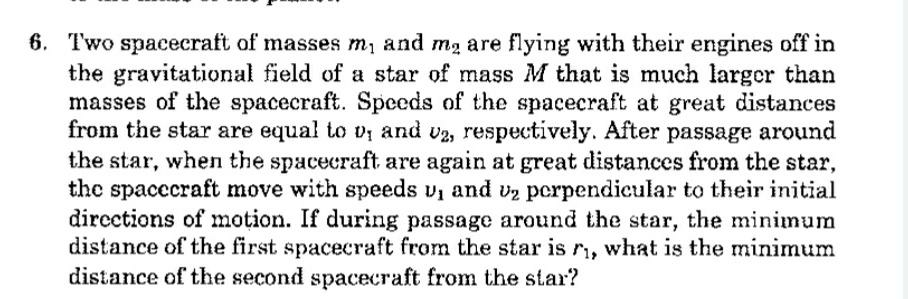# LC Problem 37Before the switch flips, the current in inductor $1$ is $\frac{E}{r}$, and the current in inductor $2$ is zero. After the switch flips, the basic equations are:

$V_C = L_1 \dot{I}_{L1} \\ V_C = L_2 \dot{I}_{L2} \\ -I_{L1} - I_{L2} = C \dot{V}_C$

Differentiating the last equation and substituting:

$-\dot{I}_{L1} - \dot{I}_{L2} = C \ddot{V}_C \\ - \frac{V_C}{L_1} - \frac{V_C}{L_2} = C \ddot{V}_C \\ \ddot{V}_C = - \Big( \frac{1}{L_1 C} + \frac{1}{L_2 C} \Big) V_C = -\omega^2 V_C$

This corresponds to SHM with angular frequency $\omega$. The general form is:

$V_C = A \sin( \omega t ) + B \cos( \omega t ) \\ \dot{V}_C = A \omega \cos( \omega t ) - B \omega \sin ( \omega t )$

Initial conditions:

$V_C (0) = 0 \\ \dot{V}_C (0) = - \frac{E}{r C}$

Applying initial conditions:

$0 = B \\ - \frac{E}{r C} = A \omega$

Finally,

$V_C = - \frac{E}{\omega r C} \sin( \omega t ) \\ \omega = \sqrt{\frac{1}{L_1 C} + \frac{1}{L_2 C}}$

So the max value of $V_C$ is:

$V_C (max) = \frac{E / (r C)}{\sqrt{\frac{1}{L_1 C} + \frac{1}{L_2 C}} }$

Knowing the expression for $V_C$, the expression for $I_{L2}$ can be calculated fairly easily by integrating:

$V_C = L_2 \dot{I}_{L2} \\ \dot{I}_{L2} = \frac{V_C}{L_2}$Note by Steven Chase
9 months, 1 week ago

This discussion board is a place to discuss our Daily Challenges and the math and science related to those challenges. Explanations are more than just a solution — they should explain the steps and thinking strategies that you used to obtain the solution. Comments should further the discussion of math and science.

When posting on Brilliant:

• Use the emojis to react to an explanation, whether you're congratulating a job well done , or just really confused .
• Ask specific questions about the challenge or the steps in somebody's explanation. Well-posed questions can add a lot to the discussion, but posting "I don't understand!" doesn't help anyone.
• Try to contribute something new to the discussion, whether it is an extension, generalization or other idea related to the challenge.
• Stay on topic — we're all here to learn more about math and science, not to hear about your favorite get-rich-quick scheme or current world events.

MarkdownAppears as
*italics* or _italics_ italics
**bold** or __bold__ bold
- bulleted- list
• bulleted
• list
1. numbered2. list
1. numbered
2. list
Note: you must add a full line of space before and after lists for them to show up correctly
paragraph 1paragraph 2

paragraph 1

paragraph 2

[example link](https://brilliant.org)example link
> This is a quote
This is a quote
    # I indented these lines
# 4 spaces, and now they show
# up as a code block.

print "hello world"
# I indented these lines
# 4 spaces, and now they show
# up as a code block.

print "hello world"
MathAppears as
Remember to wrap math in $$ ... $$ or $ ... $ to ensure proper formatting.
2 \times 3 $2 \times 3$
2^{34} $2^{34}$
a_{i-1} $a_{i-1}$
\frac{2}{3} $\frac{2}{3}$
\sqrt{2} $\sqrt{2}$
\sum_{i=1}^3 $\sum_{i=1}^3$
\sin \theta $\sin \theta$
\boxed{123} $\boxed{123}$

## Comments

Sort by:

Top Newest

@Lil Doug Here it is

- 9 months, 1 week ago

Log in to reply

@Steven Chase it was easy, Thanks

- 9 months, 1 week ago

Log in to reply

@Steven Chase how do you mention my name???

- 9 months, 1 week ago

Log in to reply

I just typed the "@" symbol, started typing your name, and let the auto complete finish it

- 9 months, 1 week ago

Log in to reply

@Steven Chase when I do this in a new note made by me, that thing doesn't work

- 9 months, 1 week ago

Log in to reply

@Steven Chase have a look in this problem, can we try analytically.- 9 months, 1 week ago

Log in to reply

I've got expressions for the velocities. It will take some time to write up, so I'll do it tomorrow.

- 9 months, 1 week ago

Log in to reply

@Steven Chase sir, two rod (one is conducting and another one not conducting) are rotating about their end points , what is the emf induced in them?

- 9 months, 1 week ago

Log in to reply

@Steven Chase whenever you have free time, take a look in this problemAs always, Thanks in advance :)

- 9 months, 1 week ago

Log in to reply

@Steven Chase I am not able to solve the 36th problem.

- 9 months, 1 week ago

Log in to reply

@Steven Chase sir I am not able to solve problem 31. :(
Sir last night you said you will write the solution of above problem (particle of charge q and mass m.............)
Still it is upto you only, because “You didn't owe me anything”
But there is still request :) Bye.

- 9 months, 1 week ago

Log in to reply

Yes, I still plan to post a solution to the damped charge problem. Probably tomorrow. It is a very tedious analytical solution

- 9 months, 1 week ago

Log in to reply

@Steven Chase are you the solver of mechanics problem (spaceship mechanics)?

- 9 months, 1 week ago

Log in to reply

@Lil Doug Hey if you dont mind me asking, which book do you get these problems from? Your latest electrostatic one, or that spaceship one?

- 9 months, 1 week ago

Log in to reply

@Aaghaz Mahajan Physics pathfinder, Irodov, Krotov .

- 9 months, 1 week ago

Log in to reply

Oooh thanks!

- 9 months, 1 week ago

Log in to reply

@Steven Chase sir is it a coincidence that when you lost a huge latex work, at that time only staff posted a new discussion for grabbing attention.?

- 9 months, 1 week ago

Log in to reply

I think it is just a coincidence

- 9 months, 1 week ago

Log in to reply

@Steven Chase you are a very lucky guy :)

- 9 months, 1 week ago

Log in to reply

Actually, that was a part 2 of the original note. So it is most likely a coincidence, that the part 1 note was filled with so many comments that a part 2 note was created exactly when Mr. Chase lost his latex.

- 8 months, 3 weeks ago

Log in to reply

@Steven Chase if you are free you can help me in 31 th problem.
In my opinion you can use pen and page also.
If you use that, staff will feel bad,you are ignoring latex due to that lost work.
So he will try to fix the problem as fast as possible. Nice trick.

- 9 months, 1 week ago

Log in to reply

I doubt it. The community section is not a high priority anymore. Almost all of the focus is on course content

- 9 months, 1 week ago

Log in to reply

@Steven Chase what does course content mean?
Does it mean the content which Brilliant provide to premium member.
I think community section should have the most priority, because a guy named mention[1247521:Steven Chase] who has exceptional skills to solve a problem is on there.

- 9 months, 1 week ago

Log in to reply

Yeah, its the subject based course content. I don't think you have to have Premium to see it though

- 9 months, 1 week ago

Log in to reply

@Steven Chase Sir I need your help in 36th problem. Please the way you use to write solutions 3 to 4 years ago like using pen and paper , then scanning the page.
Please use that technique only, it is very easy and convenient and fast to provide a solution.
Thanks in advance.
Hope I am not disturbing you.

- 9 months, 1 week ago

Log in to reply

I can upload the differential equations that describe the system, and you can take it from there. Will that work?

- 9 months, 1 week ago

Log in to reply

@Steven Chase Yes it will work :)

- 9 months, 1 week ago

Log in to reply

@Steven Chase I am. Just confused in rotating the velocity vector by $2π$ ?
How to deal with that?

- 9 months, 1 week ago

Log in to reply

×

Problem Loading...

Note Loading...

Set Loading...